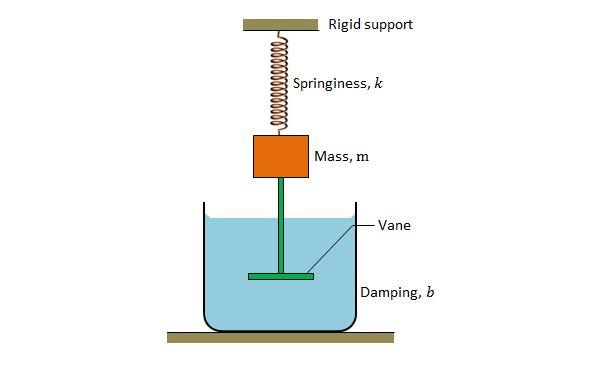Classical Mechanics

# Damping and amplitude decreasesThe above is a schematic diagram of a damped oscillator, where the block has a mass of $m=8.0\text{ kg},$ spring constant $k=130\text{ N/m},$ and damping constant $b=70\text{ g/s}.$ Approximately, how long does it take for the mechanical energy to drop to one-half its initial value?The above is a schematic diagram of a damped oscillator, where the block has a mass of $1.7\text{ kg}$ and the spring constant is $9\text{ N/m}.$ The damping force can be expressed as $-b(dx/dt),$ where $b=210\text{ g/s}.$ If the block is pulled down $12.0\text{ cm}$ and released, approximately, what is the time required for the amplitude of the resulting oscillations to fall to one-third of its initial value?The above is a schematic diagram of a damped oscillator. If the damped oscillator has mass $m=240\text{ g},$ spring constant $k=85\text{ N/m},$ and damping constant $b=70\text{ g/s},$ what is the approximate ratio of the amplitude of the damped oscillations to the initial amplitude at the end of $10$ cycles?The above is a schematic diagram of a damped oscillator, where the block has a mass of $m=250\text{ g}$ and the spring constant is $k=95 \text{ N/m}.$ It takes $4.0\text{ s}$ for the amplitude of the damped oscillations to drop to half its initial value. If we replace the block with mass $4m$ and the spring with spring constant $3k,$ how long will it take for the amplitude of the damped oscillations to drop to half its initial value?The above is a schematic diagram of a damped oscillator, where the block has a mass of $m$ and spring constant $k.$ If the ratio of the amplitude of the damped oscillations at the end of $30$ cycles to the initial amplitude is $0.57,$ what will be the ratio of the amplitude of the damped oscillations at the end of $60$ cycles to the initial amplitude?

×